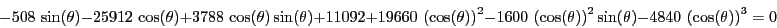Next: Solving systems with linear Up: Solving trigonometric equation Previous: Implementation   Contents

Examples

The test program Test_Solve_Trigo enable to determine the roots of any trigonometric equation which is described in a file. In this you indicate first the coefficient of the term, its sine power and then its cosine power, this for each term of the equation.

We consider the trigonometric equation derived in section 15.1.2:which has 0,5.47640186917958647 as roots. The general procedure find the roots 1.743205553711625e-11, 5.476401869153828 while the procedure using the determination of the bounds (which are [0,1.570796326794896], [5.235987755982987,6.283185307179586]) find the roots 5.974250609803587e-12, 5.476401869153828.

Jean-Pierre Merlet 2012-12-20